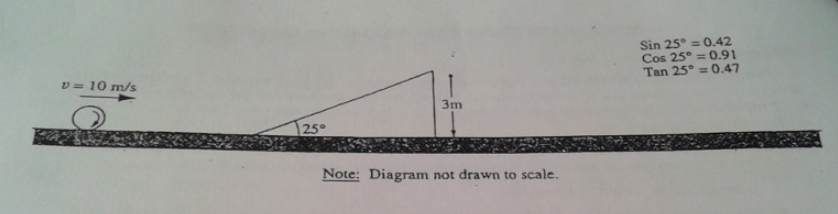# Problem: A large sphere rolls without slipping across a horizontal surface. The sphere has a constant translational speed of 10 meters per second. a mass m of 25 kilograms, and a radius r of 0.2 meter. The moment of inertia of the sphere about its center of mass is I = 2mr2/5. The sphere approaches a 25° incline of height 3 meters as shown above and rolls up the incline without slipping. A. Calculate the total kinetic energy of the sphere as it rolls along the horizontal surface. B. i. Calculate the magnitude of the sphere's velocity just as it leaves the top of the incline. ii. Specify the direction of the sphere's velocity just as it leaves the top of the incline. C. Neglecting air resistance, calculate the horizontal distance from the point where the sphere leaves the incline to the point where the sphere strikes the level surface.D. Suppose, instead, that the sphere were to roll toward the incline as stated above, but the incline were frictionless. State whether the speed of the sphere just as it leaves the top of the incline would be less than, equal to, or greater than the speed calculated in (b). Explain briefly.

###### FREE Expert Solution

Translational kinetic energy:

$\overline{){\mathbf{K}}{\mathbf{=}}\frac{\mathbf{1}}{\mathbf{2}}{{\mathbf{mv}}}^{{\mathbf{2}}}}$

Rotational kinetic energy:

$\overline{){\mathbf{K}}{\mathbf{=}}\frac{\mathbf{1}}{\mathbf{2}}{{\mathbf{I\omega }}}^{{\mathbf{2}}}}$

Relationship between v and ω:

$\overline{){\mathbf{v}}{\mathbf{=}}{\mathbf{r}}{\mathbf{\omega }}}$

Moment of inertia of a sphere:

$\overline{){\mathbf{I}}{\mathbf{=}}\frac{\mathbf{2}}{\mathbf{5}}{\mathbf{M}}{{\mathbf{R}}}^{{\mathbf{2}}}}$

A.

The sphere has both translational and rotational kinetic energy.

K = Krot + Ktrans

79% (403 ratings)###### Problem DetailsA large sphere rolls without slipping across a horizontal surface. The sphere has a constant translational speed of 10 meters per second. a mass m of 25 kilograms, and a radius r of 0.2 meter. The moment of inertia of the sphere about its center of mass is I = 2mr2/5. The sphere approaches a 25° incline of height 3 meters as shown above and rolls up the incline without slipping.

A. Calculate the total kinetic energy of the sphere as it rolls along the horizontal surface.

B.

i. Calculate the magnitude of the sphere's velocity just as it leaves the top of the incline.

ii. Specify the direction of the sphere's velocity just as it leaves the top of the incline.

C. Neglecting air resistance, calculate the horizontal distance from the point where the sphere leaves the incline to the point where the sphere strikes the level surface.

D. Suppose, instead, that the sphere were to roll toward the incline as stated above, but the incline were frictionless. State whether the speed of the sphere just as it leaves the top of the incline would be less than, equal to, or greater than the speed calculated in (b). Explain briefly.